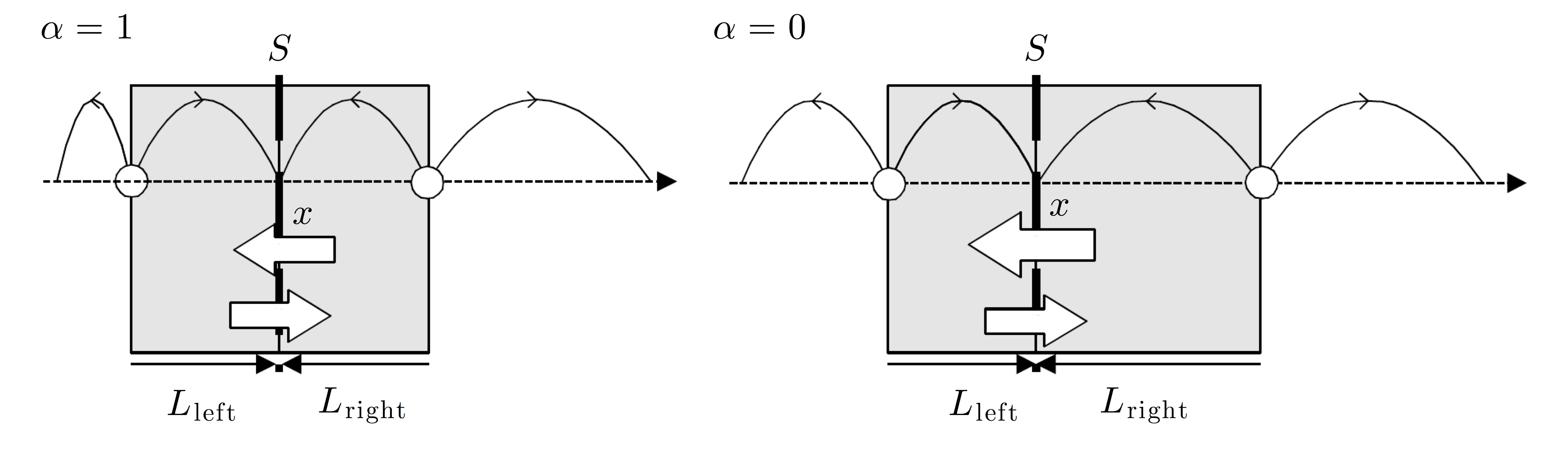# Fig. 20.4 — Spurious drift without fluxThe spurious drift, given by Eq. (20.11) with α = 1, permits one to preserve the relation between the external forces acting on the particle and the Maxwell–Boltzmann probability distribution. For example, in the absence of external forces, the Maxwell–Boltzmann probability distribution is uniform and the particle flux though any surface S must be zero (here we consider S placed perpendicular to the diffusion coefficient gradient at coordinate x). During a time interval Δt, all the particles crossing S from the left (right) are half of those included in the volume SLright (SLleft), where Lright (Lleft) is the right (left) step terminating at x taken by a walker during that time interval. Therefore, the initial particle distribution is indeed an equilibrium distribution only if the position-dependent step √2Δt in the diffusion gradient is evaluated at the final position of the step (α = 1, left cartoon). If α < 1, there is a negative flux leading to an accumulation of particles in the direction opposite to the diffusion gradient (in particular for α = 0, right cartoon). Reproduced with permission from Lanc ̧on et al., Europhys. Lett. 54, 28–34. Copyright (2001) IOP Publishing. Reproduced by permission of IOP Publishing. All rights reserved.

Fig. 20.4 — Spurious drift without flux